## 闵行区2022学年第一学期期末

•  2022/12/20
•  184 次
•  968 K

1．下面关于物理量的正负表述正确的是

A．功的正负表示大小                  B．重力势能的正负表示大小

C．电量的正负表示方向              D．电势的正负表示方向

【答案】

B

【解析】

A．功是标量，正负号不表示方向，也不表示大小，表示的是该力是动力还是阻力，故 A 错误；

B．重力势能是标量，其正负表示大小，故 B 正确；

C．电量是标量，正负号不表示方向，表示的是电性，故 C 错误；

D．电势的正负表示电势的高低，即表示的是大小，但不表示方向，故 D 错误。

2．下列式子不属于比值法定义物理量的是

A．a = $$\frac{F}{m}$$              B．E = $$\frac{F}{q}$$              C．B = $$\frac{F}{{IL}}$$            D．R = $$\frac{U}{I}$$

【答案】

A

【解析】

3．下列用国际基本单位表达电场强度的单位正确的是

A．kg·m/(C·s2)              B．kg·m/(A·s3)              C．V/m           D．N/C

【答案】

B

【解析】

4．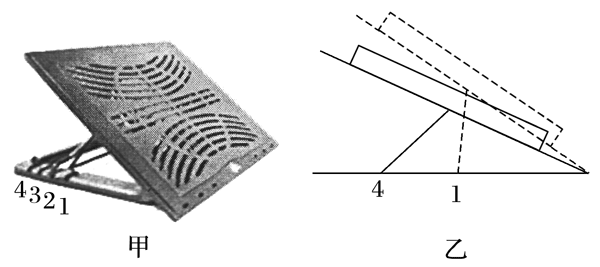笔记本电脑静止在可调节角度的底座上，如图所示，对比底座处于卡位 1 和卡位 4 时

A．卡位 4 电脑受到的摩擦力大

B．卡位 4 电脑受到的支持力小

C．底座对电脑的作用力始终不变

D．电脑受到的支持力与摩擦力大小之和等于其重力

【答案】

C

【解析】

AB．设底座与水平面的倾角为 θ，电脑的质量为 m，底座对电脑的摩擦力大小为 f，支持力大小为 N，根据受力平衡可得

N = mgcosθf = mgsinθ

C．根据平衡可知，底座对电脑的作用力大小等于电脑的重力，方向竖直向上，即底座对电脑的作用力始终不变，故 C 正确；

D．电脑受到的支持力与摩擦力大小之和为 N + f = mgcosθ + mgsinθ，不一定等于 mg，故 D 错误。

4．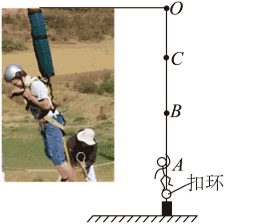如图为“反向蹦极”运动简化示意图．假设弹性轻绳的上端固定在 O 点，拉长后将下端固定在体验者身上，并通过扣环和地面固定．打开扣环，人从 A 点静止释放，沿竖直方向经 B 点上升到最高位置 C 点，B 点时速度最大．不计空气阻力，则下列说法正确的是

A．从 A 点到 C 点过程中，人的机械能一直在增大

B．从 A 点到 B 点过程中，弹性轻绳的弹性势能一直在减小

C．B 点为弹性轻绳处于原长的位置

D．从 B 点到 C 点过程中，人的机械能保持不变

【答案】

B

【解析】

AC．根据题意可知，在 B 点速度最大，则加速度为零，此时弹性轻绳的弹力等于人的重力，故此时弹性轻绳仍处于拉长状态，弹性轻绳的原长状态必处于 B、C 之间，设为 E 点，从 E 点到 C 的过程中，人做竖直上抛运动，机械能保持不变，故 A、C 错误；

B．根据 A 项的分析，从 A 点到 B 点的过程中，弹性轻绳处于拉长状态，弹力一直向上，弹力一直对人做正功，故弹性势能一直减小，故 B 正确；

D．由 A 项分析可知，从 E 点到 C 的过程中，人做竖直上抛运动，机械能保持不变，但是有 B 到 E 的过程中，弹力依然对人做正功，人的机械能是在增加的，故 D 错误。

6．电路如图所示，电源内阻为 r，L1、L2、L3 为相同规格小灯泡，当可变电阻 R 的滑片向右滑动时

A．小灯泡 L1 比 L2 亮，L2 比 L3

B．电源损耗功率增加

C．电源效率降低

D．灯泡 L2 变暗

【答案】

D

【解析】

A．由于灯泡 L2 支路的总电阻大于灯泡 L3 支路的电阻，所以灯泡 L2 支路的电流小于灯泡 L3 支路的电流，即 L2 比 L3 暗，A 错误；

B．滑动变阻器的滑片向右滑动时，接入电阻增大，干路电流减小，根据 P = I2r 可知，电源内阻损耗功率减小，B 错误；

C．根据 η = $$\frac{{{R_外}}}{{{R_外} + r}}$$ = $$\frac{1}{{1 + \frac{r}{{{R_外}}}}}$$

D．滑动变阻器的滑片向右滑动时，接入电阻增大，可分析出灯泡 L2 中的电流减小，灯泡变暗，D 正确。

7．关于“手动挡”汽车爬陡坡，下面司机的操作合理的是

A．司机只要踩油门即可，无需关心挡位是否合适

B．为了提高速度冲上陡坡，司机应该换挡位至“高速挡”

C．为增大牵引力，司机应该换挡位至“低速档”

D．司机应该换挡位至“高速挡”，并踩油门

【答案】

C

【解析】

8．图为一列简谐横波某时刻的波形图，此后 N 质点比 M 质点先回到平衡位置．下列判断正确的是

A．该简谐横波沿 x 轴正方向传播

B．此时 M 质点速度沿曲线的切线方向向上

C．此时 M 质点的速度比 N 质点的小

D．此时 M 质点的加速度比 N 质点的小

【答案】

D

【解析】

A．由于 N 质点比 M 质点先回到平衡位置，则 M 点先向上振动，由微平移法可知该简谐横波沿 x 轴负方向传播，故 A 错误；

B．M 质点振动方向向上，不是曲线切线方向，故 B 错误；

CD．由机械振动可知，离平衡位置越近，速度越大，回复力越小，加速度越小，所以 M 质点速度大于 N 质点速度，M 质点加速度小于 N 质点加速度，故选项 C 错误，D 正确。

9．某质点沿竖直方向做直线运动的位移 - 时间图像如图所示，以竖直向上为正方向，下列说法正确的是

A．该质点在 t3 时刻到达最高点

B．该质点在 0 ~ t1 时间内的速度大于在 t2 ~ t3 时间内的速度

C．在 t1 ~ t2 时间内，该质点的平均速度方向为正方向

D．在 t1 ~ t2 时间内，该质点始终处于失重状态

【答案】

D

【解析】

A．根据位移 - 时间图像可知，该质点在 t3 时刻回到出发点，故A错误；

B．位移 - 时间图像的斜率绝对值表示速度的大小，可知 0 ~ t1 时间内图像的斜率绝对值小于 t2 ~ t3 时间内的斜率绝对值，则该质点在 0 ~ t1 时间内的速度小于在 t2 ~ t3 时间内的速度，故 B 错误；

C．根据位移 - 时间图像可知，在 t1 ~ t2 时间内，质点的位移方向向下，则质点的平均速度方向向下，为负方向，故 C 错误；

D．根据位移 - 时间图像可知，在 t1 ~ t2 时间内，质点先向上做减速运动，再向下做加速运动，质点的加速度方向一直竖直向下，则该质点始终处于失重状态，故 D 正确。

10．为研究木板与物块之间的摩擦力，某同学在粗糙的长木板上放置一物块，物块通过细线连接固定在试验台上的力传感器，如图（a）。水平向左拉木板，传感器记录的 F t 图像如图（b）。下列说法中不正确的是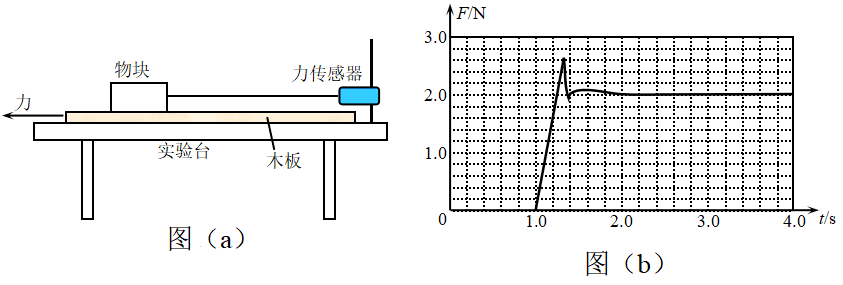A．物块受到的摩擦力方向始终水平向左

B．1.0 ~ 1.3 s 时间内，木板与物块间的摩擦力大小与物块对木板的正压力成正比

C．1.0 ~ 1.3 s 时间内，物块与木板之间的摩擦力是静摩擦力

D．2.4 ~ 3.0 s 时间内，木板可能做变速直线运动

【答案】

B

【解析】

A．木板始终向左运动或者有向左运动的趋势，可得物块对木板的摩擦力始终向右，所以物块受到木板对其的摩擦力方向始终水平向左，A 正确；

BC．根据图像可得在 1.0 ~ 1.3 s 时间内物块受到摩擦力在变化，所以可得此时为静摩擦力，此时的静摩擦力大小与物块对木板的正压力无关，B 错误，C 正确；

D．2.4 ~ 3.0 s 时间内传感器示数不再变化说明此时摩擦力已经变为滑动摩擦力，此时木板只要运动就行，可能做变速直线运动，D 正确。

11．如图所示，AB、CD 为一圆的两条直径且相互垂直。O 点为圆心，空间存在一未知静电场，方向与圆周所在平面平行。现有一电子，在电场力作用下，先从 A 点运动至 C 点，动能减少了 E；又从 C 点运动到 B 点，动能增加了 E，那么

A．A 到 C 电场力做正功，C 点电势 φC 高于 A 点 φA

B．C 到 B 电场力做正功，C 点电势 φC 高于 B 点 φB

C．该电场可能是匀强电场，电场线方向为 D 指向 C

D．该电场可能为正点电荷电场，且电荷处于 O 点

【答案】

C

【解析】

A．从 A 点运动至 C 点动能减少，所以电场力做负功；电势能增加，因为是电子带负电，所以可得 C 点电势 φC 低于 A 点 φA，A 错误；

B．同理 C 到 B 电场力做正功，动能增加，电势能减小，可得 C 点电势 φC 低于 B 点φB，B 错误；

C．根据前面分析可得 A、B 点电势能相同，电势相等，若是匀强电场，电场线垂直 AB 连线，又因为 C 点电势低于 A、B 两点，沿着电场线方向电势降低所以方向为 D 指向 C，C 正确；

D．若该电场为正点电荷电场，且电荷处于 O 点，可知 A、C 两点电势相同与题意不符，D 错误。

12．一圆形线圈与一均匀的扁平条形磁铁同在一平面内，磁铁中央与圆心 O 重合．为了在磁铁开始运动时在线圈中得到一方向如图所示的感应电流 i，磁铁的运动方式应为

A．使磁铁在线圈平面内绕 O 点沿逆时针方向转动

B．使磁铁沿垂直于线圈平面的方向向纸外做平动

C．使磁铁沿垂直于线圈平面的方向向纸内做平动

D．N 极向纸内，S 极向纸外，使磁铁绕 O 点转动

【答案】

D

【解析】

A．使磁铁在线圈平面内绕 O 点沿逆时针方向转动，穿过线圈的磁通量保持不变为零，不会产生感应电流，故 A 错误；

BC．使磁铁沿垂直于线圈平面的方向向纸外或向内做平动时，穿过线圈的磁通量仍然保持不变为零，不会产生感应电流，故 BC 错误；

D．图示时刻穿过线圈的磁通量为零，当 N 极向纸内，S 极向纸外，使磁铁绕 O 点转动时，使得穿过线圈的磁通量垂直纸面向里增大，根据楞次定律，可判断知在线圈中产生的感应电流为逆时针方向，故 D 正确。

13．用 2×106 Hz的超声波检查胆结石，该超声波在结石和胆汁中的波速分别为 2 250 m/s 和 1 500 m/s，则该超声波在结石中的波长是胆汁中的______倍。用超声波检查胆结石是因为超声波的波长较短，遇到结石时_______（选填“容易”或“不容易”）发生衍射。

【答案】

1.5，不容易

【解析】

14．在高度 180 m 处有两只气球（气球 a 和气球 b），以相同速度 10 m/s 分别匀速上升和匀速下降，此时，在这两只气球上各掉出一物体，则这两个物体落到地面时的时间差_______s，所经过的路程差_________m。（物体空气阻力不计）

【答案】

2，10

【解析】

（1）根据题意可得气球 a 上掉出的物体先做竖直上抛运动，当回到初始位置时以同样大小的速度向下做匀加速直线运动，气球 b 掉出的物体从掉出后就开始向下做匀加速直线运动，所以可得两个物体落到地面时的时间差即为气球 a 上掉出的物体从掉出到回到掉出位置时的时间，即 Δv = 2×$$\frac{v}{g}$$ = 2×$$\frac{10}{10}$$ s = 2 s

（2）同理所经过的路程差为气球 a 上掉出的物体从掉出到回到掉出位置时经过的路程，即

Δs = 2×$$\frac{{{v^2}}}{{2g}}$$ = 2×$$\frac{{{10^2}}}{{2 \times 10}}$$ m = 10 m

【答案】

【解析】

（1）设地球质量为 M，天舟五号货运飞船质量为 m，运行半径为 r，根据万有引力提供向心力可得 $$\frac{{GMm}}{{{r^2}}}$$ = m$$\frac{{{v^2}}}{r}$$ 解得 v = $$\sqrt {\frac{{GM}}{r}}$$

（2）组合体的角速度约为 ω = $$\frac{{2\pi }}{T}$$ = $$\frac{{2\pi }}{{90 \times 60}}$$ rad/s = 1.16×10−3 rad/s

16．如图，倾角为 θ 的光滑斜面固定在水平地面，与斜面平行的轻质绝缘细线上端固定在斜面顶端，下端系质量为 m、电荷量为 q 的小球 A。A 右侧等高处固定带等量同种电荷的小球 B，若 AB 间距为 $$\sqrt {\frac{{k{q^2}}}{{mg\tan \theta }}}$$，则 B 在 A 处激发的电场强度大小是__________，斜面受到 A 的压力大小为_________。（A、B 可视为点电荷）

【答案】

$$\frac{{mg\tan \theta }}{q}$$，$$\frac{{mg}}{{\cos \theta }}$$

【解析】

（1）由点电荷场强公式可得

E = $$\frac{{kq}}{{{{\left( {\sqrt {\frac{{k{q^2}}}{{mg\tan \theta }}} } \right)}^2}}}$$（2）小球 A 受力分析如图所示。小球所受合力为零，垂直斜面方向

N = mgcosθ + Eqsinθ = mgcosθ + mgtanθsinθ = $$\frac{{mg}}{{\cos \theta }}$$

17．四根平行的通电长直导线，其横截面恰好在一个边长为 L 的正方形的四个顶点上，电流方向如图，其中 A、C 导线中的电流大小为 I1，B、D 导线中的电流大小为 I2。若通电长直 导线产生的磁场中某点的磁感应强度满足 B = k  $$\frac{I}{r}$$（其中 k 为比例系数，I 为电流强度，r 为该点到直导线的距离），kI1I2L 为已知量，则正方形中心 O 处的磁感应强度大小为_________。若 A 导线所受的磁场力恰好为零，则 I1I2 =_________。

【答案】

0，2∶1

【解析】

（1）根据磁场的矢量合成法则，B、D 导线在 O 处的合磁感应强度为零，A、C 导线在 O 处的合磁感应强度也为零，所以 O 处的磁感应强度大小为 0。

（2）B 导线在 A 处的磁场方向竖直向下，D 导线在A处的磁场方向水平向左，可得 B、D 导线在 A 处的合磁感应大小为 B1 = $$\sqrt 2$$ k $$\frac{{{I_2}}}{L}$$

C 导线在 A 处的磁感应大小为 B2 = k  $$\frac{{{I_1}}}{{\sqrt 2 L}}$$

18．如图（a）所示为“用 DIS 测电源的电动势和内电阻”的部分实验电路图，电流传感器有微小电阻，图乙是某同学实验中得到的 U – I 图线。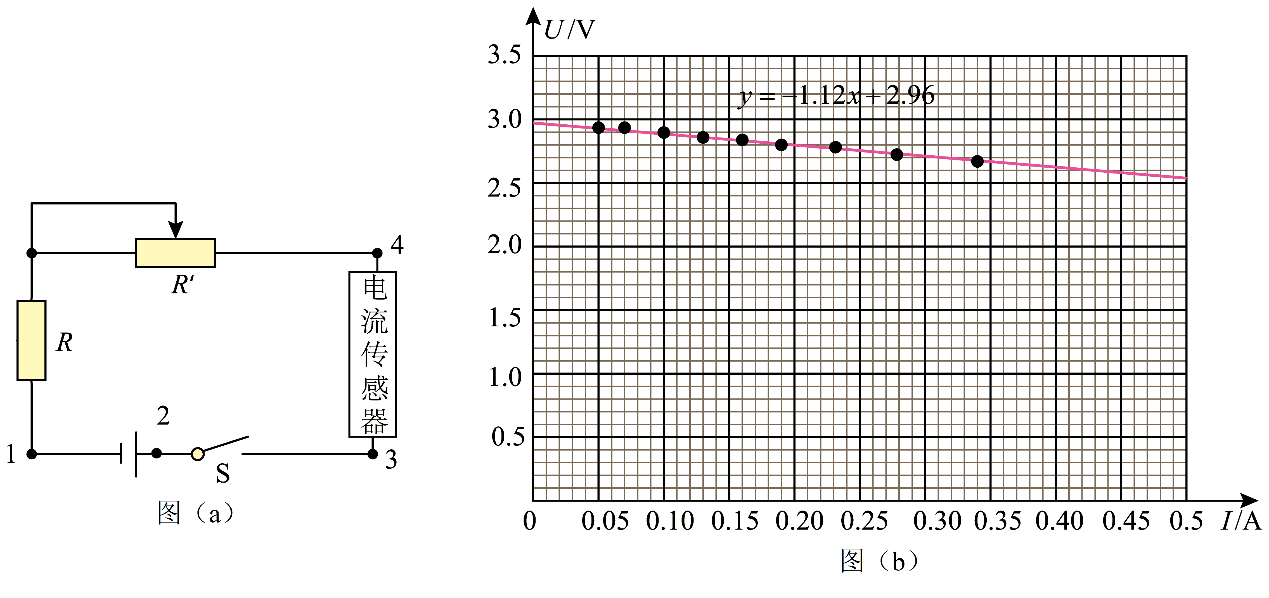（1）实验中还需要_________传感器，其接线应接在图（a）电路中的“1”和_______位置（“2、3、4”中选填）；

（2）由图（b）实验图线的拟合方程 y = − 1.12x + 2.96 可得，该电源电动势 E = _________V，内阻r = _________Ω；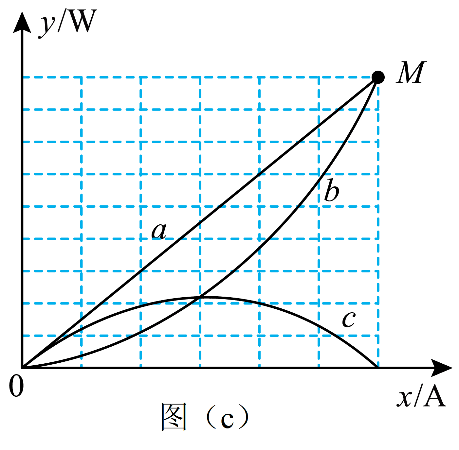（3）根据实验测得的 IU 数据，若令 y = IUx = I 则由计算机拟合得出的 y x 图线应是图（c）中的________（选填“a”、“b”或“c”），其余两条图线分别是令 y = IEy = I2r 得出的；

（4）由第（2）问中得到的电源电动势和内阻的值，推测图丙中 M 点的坐标为___________。（保留到小数点后面2位）

【答案】

（1）电压，3

（2）2.96，1.12

（3）c

（4）（2.64，7.82）

【解析】

（1）实验中还需要电压传感器测路端电压。开关要起到作用，所以不能接在 2；电流传感器有微小电阻，要正确测量端电压，不能接在 4。电压传感器接线应分别接在图（a）电路中 1 和 3 位置。

（2）根据闭合电路欧姆定律 U = EIr。由图（b）实验图线的拟合方程可得，图像纵坐标的截距表示电源电动势，则有 E = 2.96 V，斜率的绝对值表示电源的内阻，则有 r = 1.12 Ω。

（3）若令 y = IUx = I 则由计算机拟合得出的 yx 图线应是电源的输出功率与干路电流的关系，由于电源的输出功率随外电阻的增大是先增大后减小，当外电阻等于电源内阻时输出功率最大，所以该图线应是 c。

（4）M 是电路的状态是外电阻为 0，此时 ym = $$\frac{{{E^2}}}{r}$$ = $$\frac{{{2.96^2}}}{1.12}$$ W ≈ 7.82 W；xm = $$\frac{E}{r}$$ = $$\frac{2.96}{1.12}$$ A ≈ 2.64 A。所以推测图（丙）中 M 点的坐标为（2.64，7.82）。

19．如图（a）所示，一根足够长的细杆与水平成 θ = 37° 固定，质量为 m = 1 kg 的小球穿在细杆上静止于细杆底端 O 点，水平向右的 F 作用于小球上，经时间 t1 = 0.2 s 后停止作用，小球沿细杆运动的部分 v t 图像如图（b）所示（取 g = 10 m/s2，sin37° = 0.6，cos37° = 0.8）。求：（1）小球在 0 ~ 0.2 s 内的加速度 a1 和 0.2 ~ 0.4 s 内的加速度 a2

（2）小球与细杆之间的动摩擦因数 μ

（3）0 ~ 0.2 s 内水平作用力 F 的大小；

（4）试分析小球是否可以返回 O 点？说明原因。

【答案】

（1）a1 = 20 m/s2a2 = − 10 m/s2

（2）μ = 0.5

（3）F = 60 N

（4）能够返回到 O 点

【解析】

（1）根据 a = $$\frac{{\Delta v}}{{\Delta t}}$$，a1 = 20 m/s2a2 = − 10 m/s2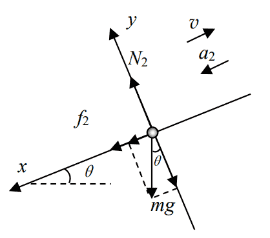（2）0.2 ~ 0.4 s，小球受力如图：

mgsin37° + μN2 = ma2

N2mgcos37° = 0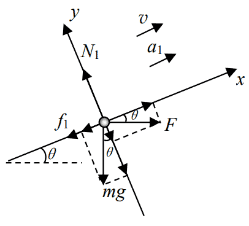（3）0 ~ 0.2 s，小球受力如图：

Fcos37° − mgsin37° − μN1 = ma1

N1mgcos37° + Fsin37° = 0

（4）mgsinθ = 6 N > f静max = μmgcosθ = 4 N，小球上升到最高点后会沿杆加速下滑

20．发电机和电动机具有装置上和机理上的类似性。直流发电机和直流电动机的工作原理可以简化为如图（a）、图（b）所示的情景。（1）求图（a）、图（b）中，金属棒两端 a、b 两点间的电压；

（2）求在 Δt 时间内，图（a）“发电机”产生的电能 E1 和图（b）“电动机”输出的机械能 E2

（3）分析 Δt 时间内，图（a）和图（b）中能量转化的过程。

【答案】

（1）图（a）：Uab = $$\frac{{BLvr}}{{R + r}}$$

（2）图（a）：E1 = $$\frac{{{B^2}{L^2}{v^2}}}{{R + r}}$$ Δt

（3）图（a）：外界能量 E1，通过外力 F 做功，进入系统；通过克服 FA 做功转化为电能；通过电流做功转化为 Rr 上的焦耳热。

【解析】

（1）图（a）： E = Blv

I = $$\frac{{BLv}}{{R + r}}$$

Uab = Ir = $$\frac{{BLvr}}{{R + r}}$$

Uab = IR + BLv

（2）图（a）：E1 = EIΔt = BLv·$$\frac{{BLv}}{{R + r}}$$·Δt = $$\frac{{{B^2}{L^2}{v^2}}}{{R + r}}$$ Δt

E2 = BILvΔt

（3）图（a）：ab 棒受力如图，因为匀速，F = FA

2006 - 2023，推荐分辨率1024*768以上，推荐浏览器Chrome、Edge等现代浏览器，截止2021年12月5日的访问次数：1872万9823 站长邮箱